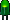• Home• Patreon• Links• Books
Number Converting:

# 3. Decimal Number Conversion

(article continued from previous page)

A repeated division and remainder algorithm can convert decimal to binary, octal, or hexadecimal.

1. Divide the decimal number by the desired target radix (2, 8, or 16).
2. Append the remainder as the next most significant digit.
3. Repeat until the decimal number has reached zero.

## Decimal to Binary

Here is an example of using repeated division to convert 1792 decimal to binary:

 Decimal Number Operation Quotient Remainder Binary Result 1792 ÷ 2 = 896 0 0 896 ÷ 2 = 448 0 00 448 ÷ 2 = 224 0 000 224 ÷ 2 = 112 0 0000 112 ÷ 2 = 56 0 00000 56 ÷ 2 = 28 0 000000 28 ÷ 2 = 14 0 0000000 14 ÷ 2 = 7 0 00000000 7 ÷ 2 = 3 1 100000000 3 ÷ 2 = 1 1 1100000000 1 ÷ 2 = 0 1 11100000000 0 done.

### Decimal to Octal

Here is an example of using repeated division to convert 1792 decimal to octal:

 Decimal Number Operation Quotient Remainder Octal Result 1792 ÷ 8 = 224 0 0 224 ÷ 8 = 28 0 00 28 ÷ 8 = 3 4 400 3 ÷ 8 = 0 3 3400 0 done.

Here is an example of using repeated division to convert 1792 decimal to hexadecimal:

 Decimal Number Operation Quotient Remainder Hexadecimal Result 1792 ÷ 16 = 112 0 0 112 ÷ 16 = 7 0 00 7 ÷ 16 = 0 7 700 0 done.

The only addition to the algorithm when converting from decimal to hexadecimal is that a table must be used to obtain the hexadecimal digit if the remainder is greater than decimal 9.

 Decimal: 0 1 2 3 4 5 6 7 Hexadecimal: 0 1 2 3 4 5 6 7
 Decimal: 8 9 10 11 12 13 14 15 Hexadecimal: 8 9 A B C D E F

The addition of letters can make for funny hexadecimal values. For example, 48879 decimal converted to hex is:

 Decimal Number Operation Quotient Remainder Hexadecimal Result 48879 ÷ 16 = 3054 15 F 3054 ÷ 16 = 190 14 EF 190 ÷ 16 = 11 14 EEF 11 ÷ 16 = 0 11 BEEF 0 done.